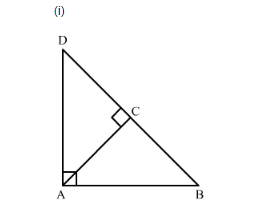# ∆ABD is a right triangle right-angled at A and AC ⊥ BD. Show that

Question:

$\triangle \mathrm{ABD}$ is a right triangle right-angled at $\mathrm{A}$ and $\mathrm{AC} \perp \mathrm{BD}$. Show that

(i) $\mathrm{AB}^{2}=\mathrm{BC} \cdot \mathrm{BD}$

(ii) $\mathrm{AC}^{2}=\mathrm{BC} . \mathrm{DC}$

(iii) $\mathrm{AD}^{2}=\mathrm{BD} \cdot \mathrm{CD}$

(iv) $\frac{\mathrm{AB}^{2}}{\mathrm{AC}^{2}}=\frac{\mathrm{BD}}{\mathrm{DC}}$

Solution:In $\triangle A B D$ and $\triangle A B C$,

$\angle A C B=\angle A=90^{\circ}$

$\angle B=\angle B$ (Common angle)

So, by AA criterion $\triangle A B D \sim \triangle C B A$

$\therefore \frac{A B}{B C}=\frac{B D}{A B}=\frac{A D}{A C}$

$\therefore \frac{A B}{B C}=\frac{B D}{A B}$

$\therefore A B^{2}=B D \cdot B C$....(1)

(ii) In $\triangle A B D$ and $\triangle A C D$,

$\angle C=\angle A=90^{\circ}$

$\angle D=\angle D \quad$ (Common angle)

So, by AA criterion $\triangle A B D \sim \triangle C A D$

$\therefore \frac{A B}{A C}=\frac{B D}{A D}=\frac{A D}{C D}$

$\therefore \frac{B D}{A D}=\frac{A D}{C D}$

$\therefore A D^{2}=B D \cdot C D$....(2)

(iii) We have shown that $\triangle A B D$ is similar to $\triangle C B A$ and $\triangle A B D$ is similar to $\triangle C A D$ therefore, by the property of transitivity $\triangle C B A$ is similar to $\triangle C A D$.

$\therefore \frac{B C}{A C}=\frac{A B}{A D}=\frac{A C}{C D}$

$\therefore \frac{B C}{A C}=\frac{A C}{C D}$

$\therefore A C^{2}=B C \cdot C D$....(3)

(iv) Now to obtained $\mathrm{AB}^{2} / \mathrm{AC}^{2}=\mathrm{BD} / \mathrm{DC}$, we will divide equation (1) by equation (2) as shown below,

$\therefore \frac{A B^{2}}{A C^{2}}=\frac{B D \cdot B C}{B C \cdot C D}$

Canceling BC we get,

$\frac{A B^{2}}{A C^{2}}=\frac{B D}{C D}$

Therefore, $\frac{A B^{2}}{A C^{2}}=\frac{B D}{C D}$